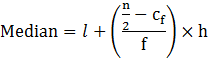Guru

# The following frequency distribution gives the monthly consumption of an electricity of 68 consumers in a locality. Find the median, mean and mode of the data and compare them. Q.1

• 0

How i solve the problem of question no.1 of Statistics of exercise 14.3 of ncert class 10th solution, how i solve this problem in easy and simple way give me the best way to solve this problem The following frequency distribution gives the monthly consumption of an electricity of 68 consumers in a locality. Find the median, mean and mode of the data and compare them.

Share

1. From the table, it is observed that, n = 68 and hence n/2=34

Hence, the median class is 125-145 with cumulative frequency = 42

Where, l = 125, n = 68, Cf = 22, f = 20, h = 20

Median is calculated as follows:=125+((34−22)/20) × 20

=125+12 = 137

Therefore, median = 137

To calculate the mode:

Modal class = 125-145,

f1=20, f0=13, f2=14 & h = 20

Mode formula:

Mode = l+ [(f1-f0)/(2f1-f0-f2)]×h

Mode = 125 + ((20-13)/(40-13-14))×20

=125+(140/13)

=125+10.77

=135.77

Therefore, mode = 135.77

• 0